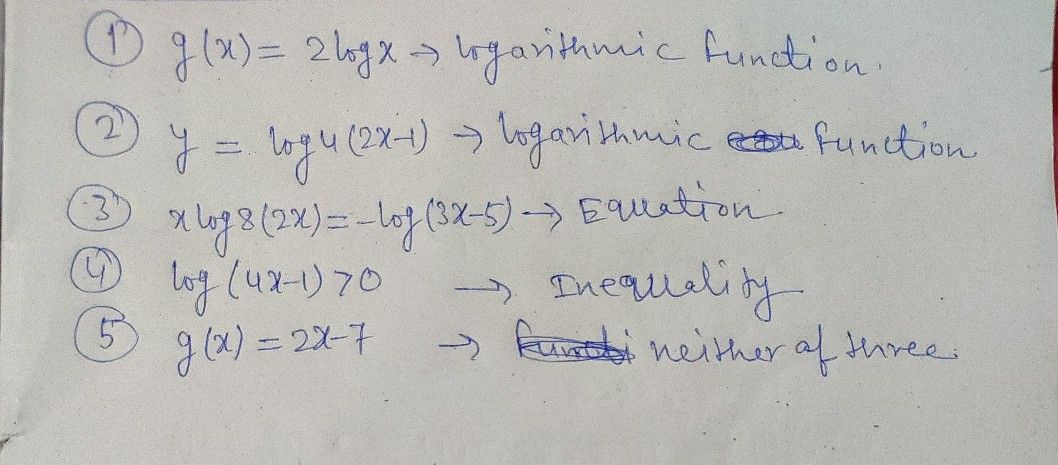Symbol
ProblemActivity $1$ Determine whether each $01$ the given below $1s$ $a$ logarithmic function, $2$ logarithmic equation, a logarithmic inequality, or neither of the three. Enjoy working while recalling your previous lessons'regarding logarithm. Have fun! 1. gtx) = 2logx $2$ $y$ $-$ $log4\left(2x-1\right)$ $\left(2x\right)=-log\left(3x-5\right)$ $3$ xlog8(2x) 4. $log\left(+x-1\right)>0$ $5$ g(x) $=2x-7$ How did you distinguish logarithmic functions, logarithmic equations and logarithmic inequalities from each other?
10th-13th grade
Other
Search count: 120
SolutionQanda teacher - Ranjeet